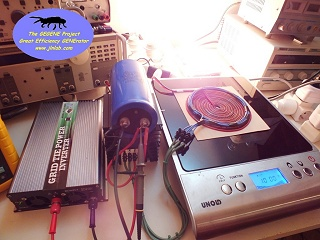TEST #15 : Additional measurements with the GEGENE v1.3
created on december 28, 2012 - JLN Labs - last update january 17, 2013
All informations and diagrams are published freely (freeware) and are intended for a private use and a non commercial use.
Toutes les informations et schémas sont publiés gratuitement ( freeware ) et sont destinés à un usage personnel et non commercial

Cliquez ici pour la version FRANCAISE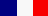January 17, 2013 - TEST #15 : Here is a new serie of additional measurements to the TEST#14 on the GEGENE v1.3. The purpose of this test is to improve the accuracy of the current measurements via the non inductive 0.1 Ohm resistance (Mundorf MR10 10W) by removing all potential risks which may interfere with the measurement.

In the previous configuration used in the TEST#14, the Ch2 probe of the oscilloscope is connected accross the 0.1 Ohm resistor. The length of this resistor is 50 mm, so there is a possibility that the voltage measurement can be jammed by the magnetic field flowing around this resistor. This magnetic field around the resistance may eventually induce an additional parasitic voltage which can trouble the measurement. Below, a diagram which shows the effect of the magnetic field surrounding the resistance on the measurement loop of the Ch2 probe. Thanks to zgreudz for his relevant remark about this :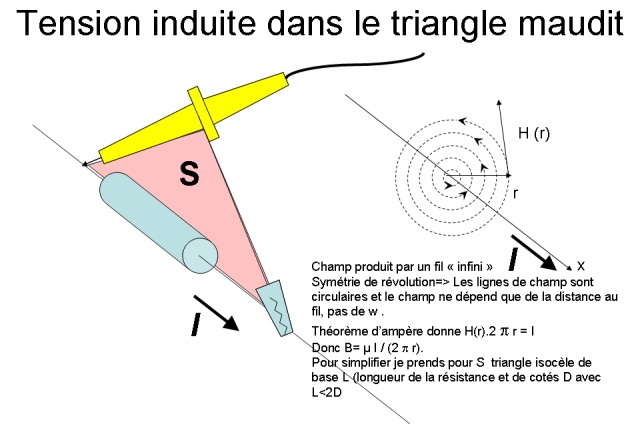To check if the presence of the triangular loop (the pink surface) is able to create trouble in the current measurement, I have built a new setup for the measurement of the current so as to reduce this surface near to zero and thus to nullify the electromagnetic interference produced by the magnetic field surrounding the resistance. Here this new setup :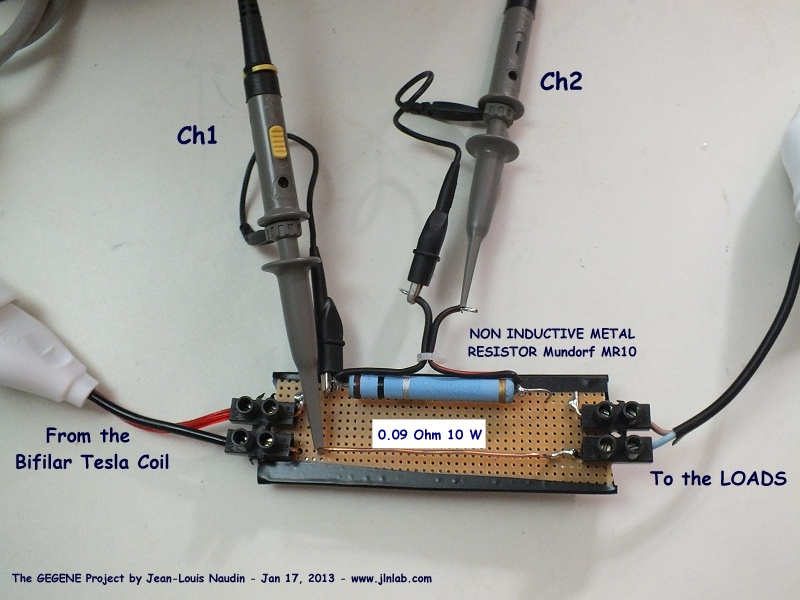In this setup, the influence of the magnetic field surrounding the resistance is negligible.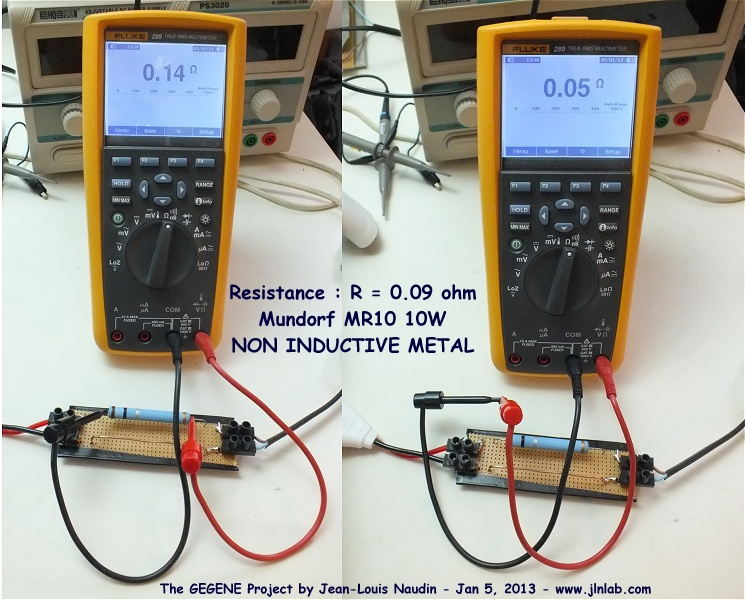The exact value of the resistance is 0.09 Ohm.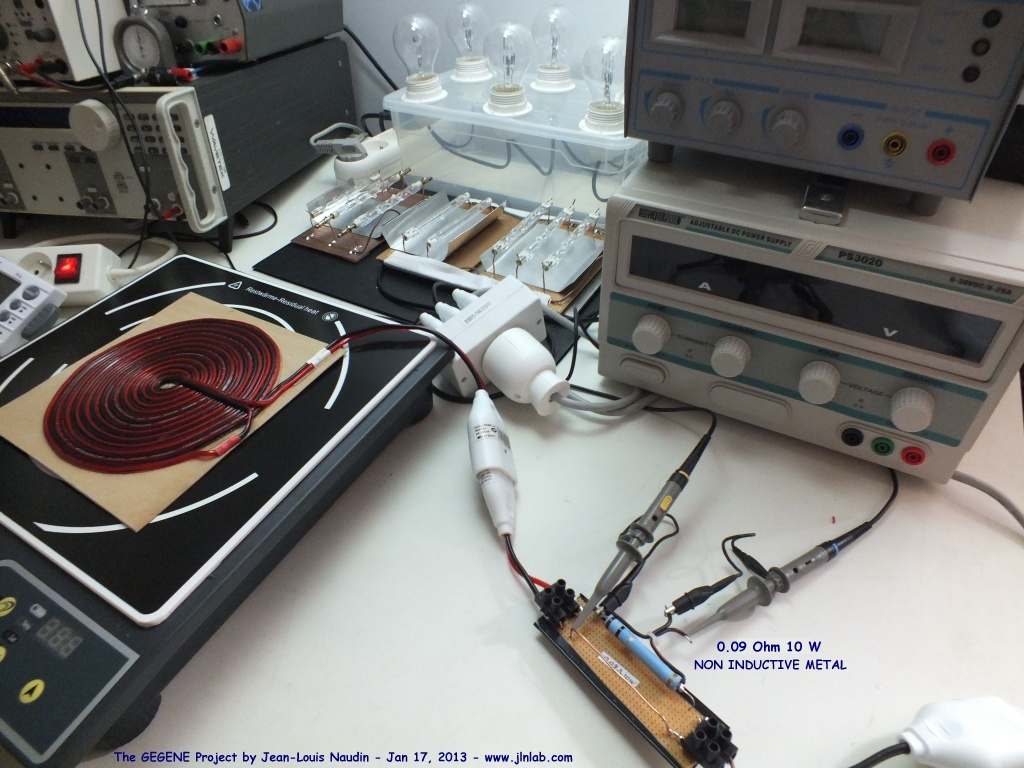Here the new tests results on the TEST#15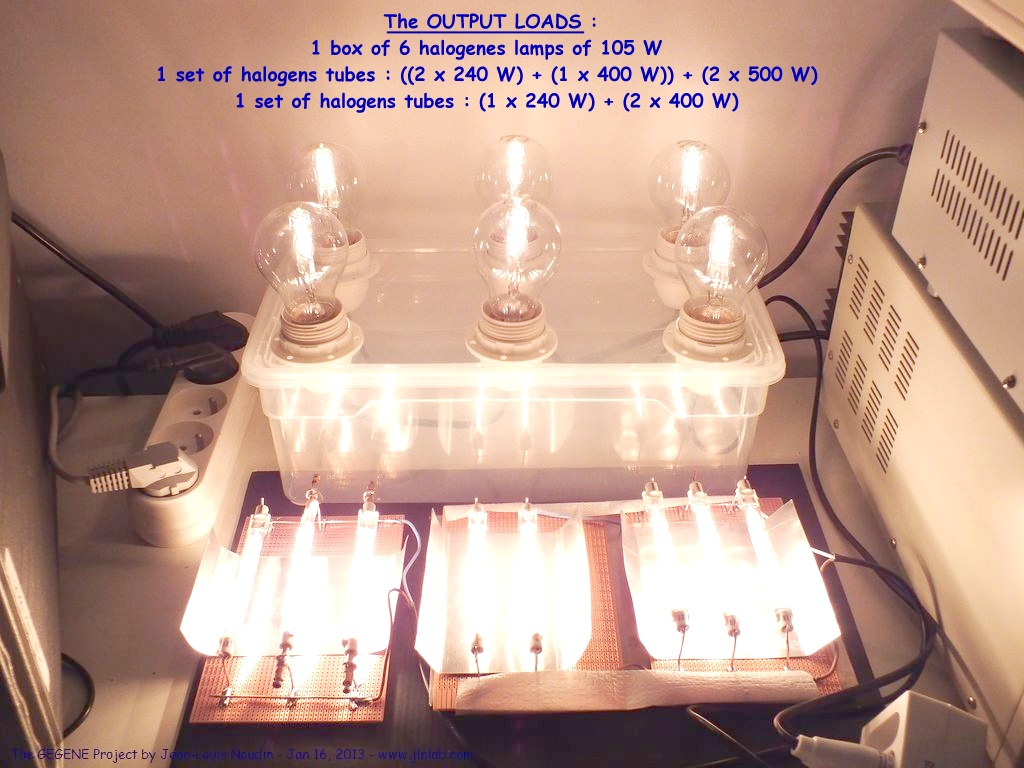I have used the same loads at the GEGENE v1.3 output than in the TEST#14 : for a total of 3550 W of halogen lamps.

In this test, the output power has been limited to the level 5/10 i.e. at the middle power (about 1200 W).

To measure the voltage and the current at the output for powering the halogen lamps, the two scope probes are set to X10 and are connected to the flat bifilar coil output. The probe Ch1 is used to measure the voltage accross the flat bifilar coil output and the probe Ch2 is used to measure the current i.e. the voltage accross a 0.1 Ohm non inductive resistor Mundorf MR10 10W. Then the datas are sent to a datasheet to compute true RMS values and the efficiency. The electrical power input of the induction cooker is measured with a Wattmeter Energy Logger 4000F directly connected on the power grid.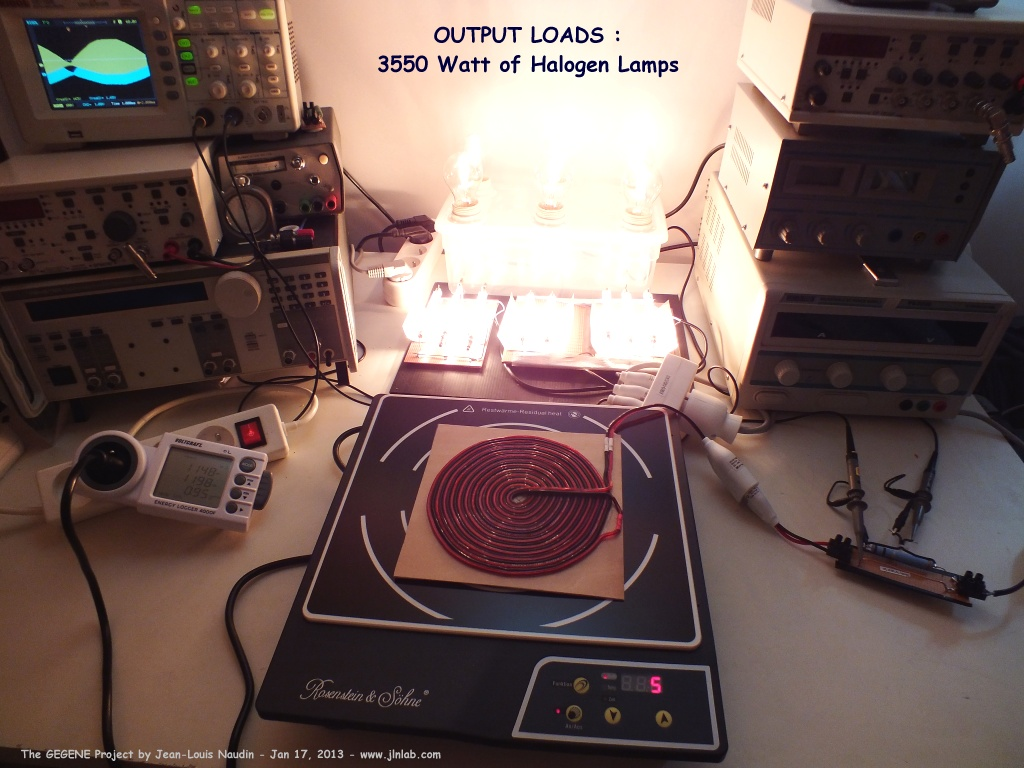The electrical power for the induction cooker is measured with a Wattmeter Energy Logger 4000F directly connected on the power grid :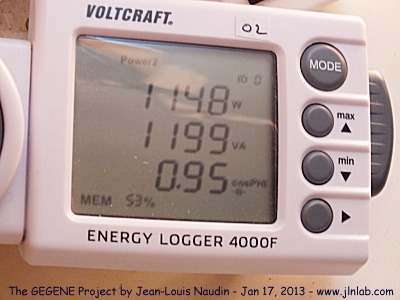The Wattmeter measures 1148 Watt at the INPUT of the induction cooker.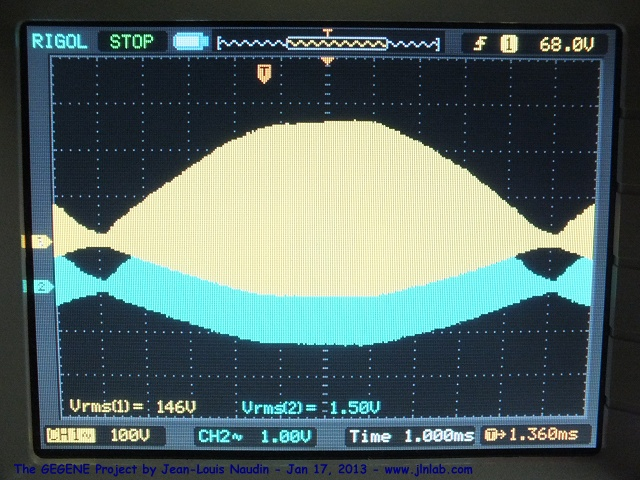Above the True RMS voltage measured by the Rigol digital oscilloscope.

So as to get a good accuracy of the measured values for the mathematical computation, the sampling rate has been set to 20.00 MSa/s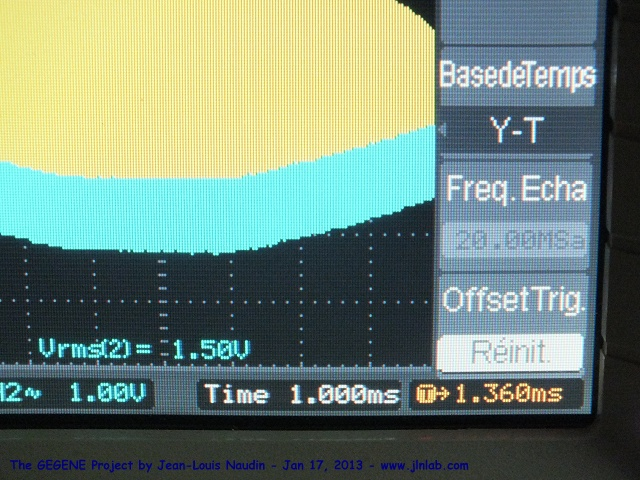We can observe that there is no phase shift between the voltage and the current measured.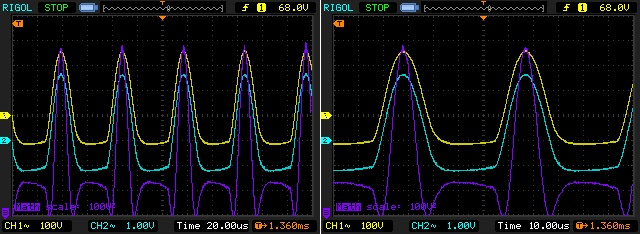Below, the scope datas computed with GNU Octave 3.2.4 (freeware and full compatible version of MATLAB)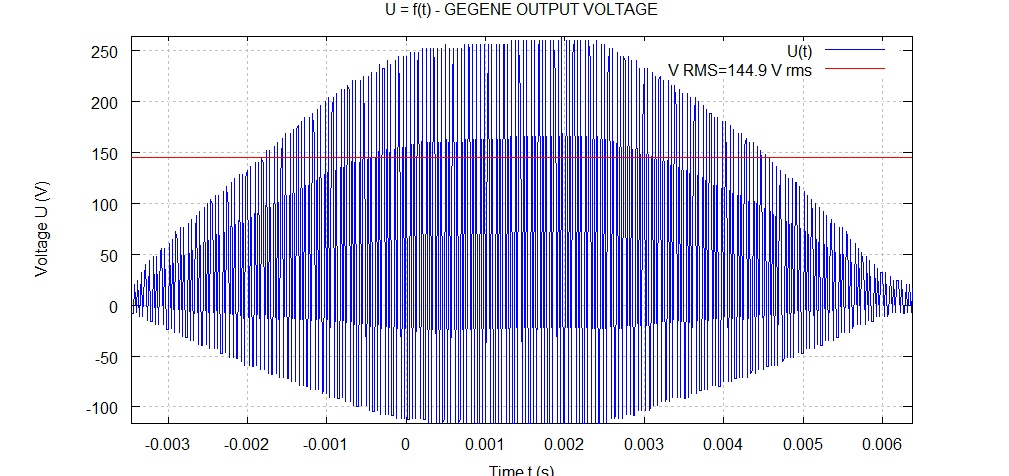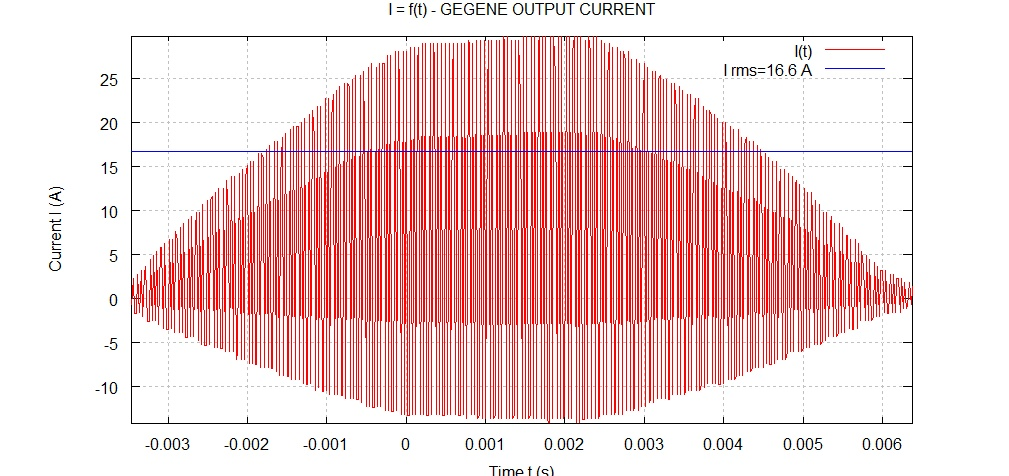We can also observe that
the computed RMS current is very close to the measured value in real time by the digital oscilloscope...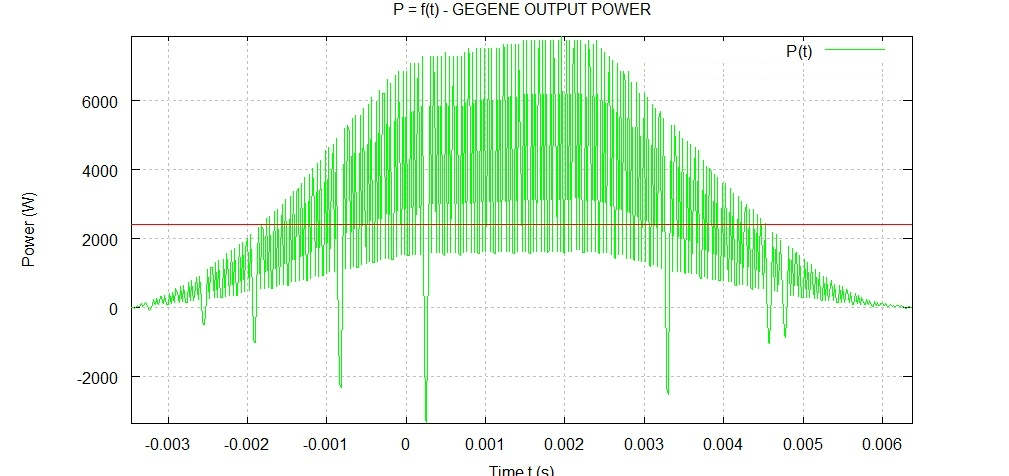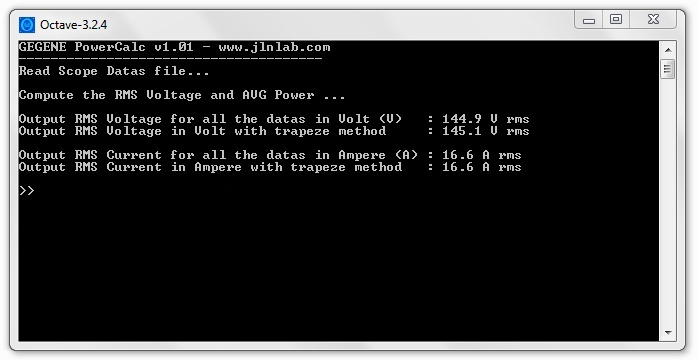Under Octave launch power_calc

Comments : According to the results of this TEST#15, we can say that the electromagnetic disturbance on the Ch2 by the magnetic field surrounding the resistor is really negligible on the measurements and the computations of the power output of the GEGENE.

NEXT TEST :

Stay tuned,

Email: jnaudin509@aol.com